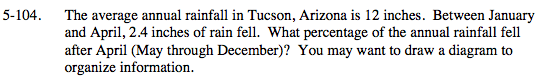### Home > MC2 > Chapter 5 > Lesson 5.3.2 > Problem5-104

5-104.If 2.4 inches of rain fell between January and April and the annual rainfall is about 12 inches,
the remainder must have fallen from May through December.

Since 12 − 2.4 = 9.6, the rainfall from May through December must be 9.6 inches.
If 9.6 inches is taken as a fraction of the total average annual rainfall of 12 inches,
what percentage of the annual rainfall after April does this fraction represent?

$\frac{9.6}{12} \rightarrow \frac{96}{120} \rightarrow \frac{4}{5}$